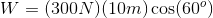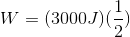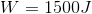# SAT II Physics : Work

## Example Questions

### Example Question #8 : Calculating Work

A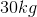crate is pushed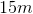across the floor. If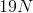of force was used to achieve this motion, how much work was done?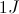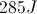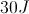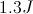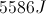Explanation:

The formula for work is: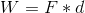Given the values for force and distance, we can calculate the work done.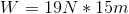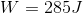Note that no work is done by the force of gravity or the weight of the box, since the vertical position does not change.

### Example Question #1 : Work

A man moves a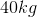crate by pulling a rope at a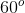angle with the ground and a constant force of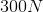. How much work has the man performed after pulling the crate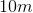?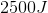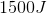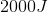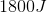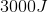Explanation:

Work is given by the equation: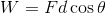We know the force with which the man pulls, the angle, and the distance that the crate travels. Using these values we can solve the equation for the work done.